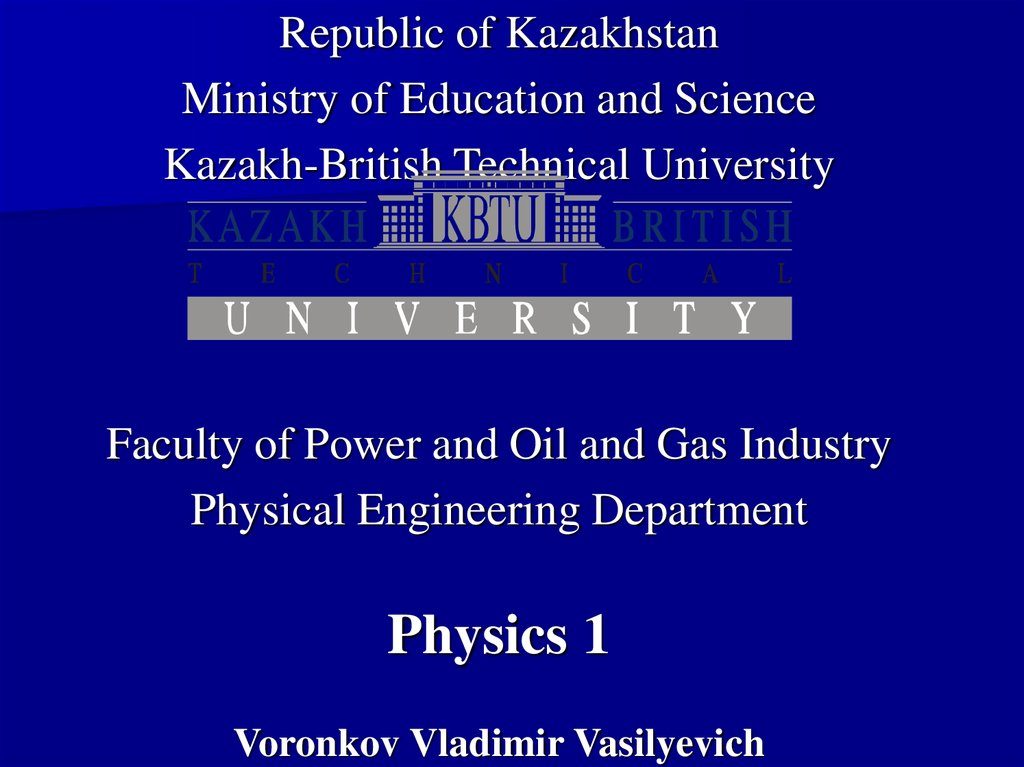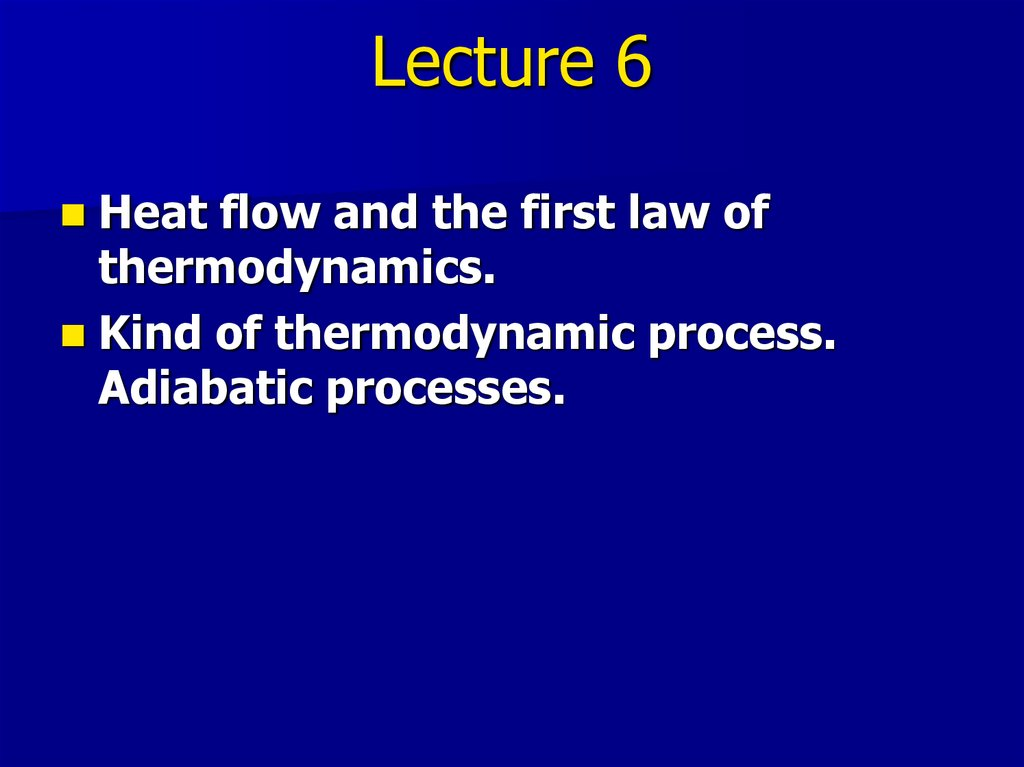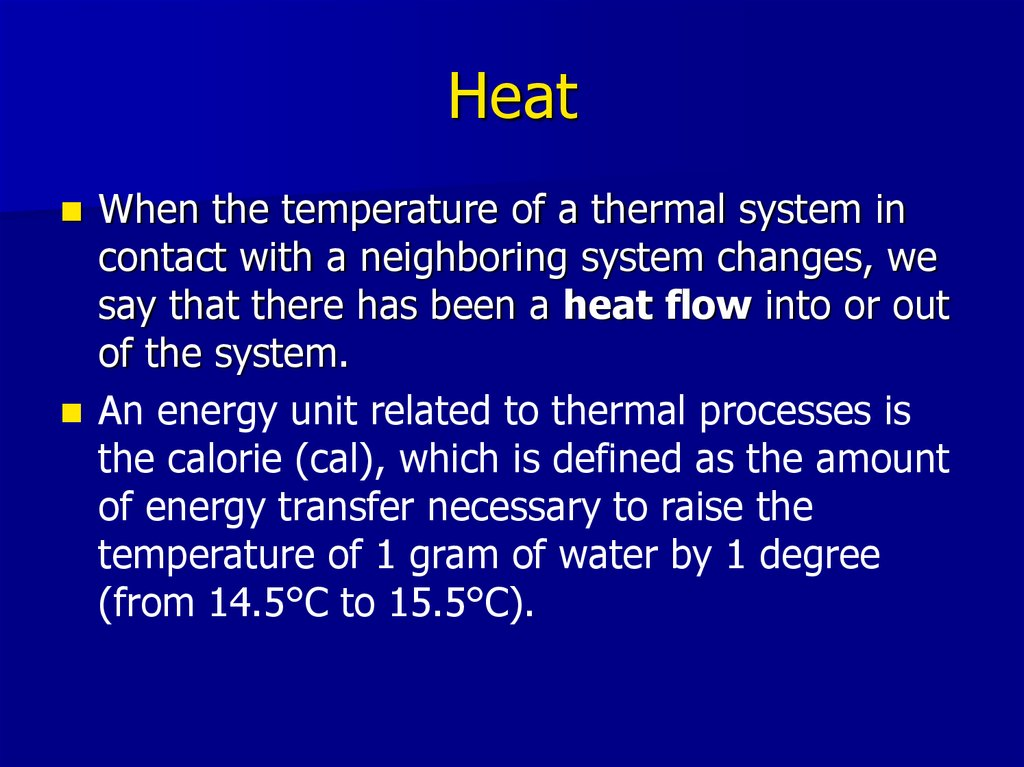# Heat flow and the first law of thermodynamics. Kind of thermodynamic process. Adiabatic processes

## 1.

Republic of Kazakhstan
Ministry of Education and Science
Kazakh-British Technical University
Faculty of Power and Oil and Gas Industry
Physical Engineering Department
Physics 1

## 2. Lecture 6

Heat flow and the first law of
thermodynamics.
Kind of thermodynamic process.

## 3. Heat

When the temperature of a thermal system in
contact with a neighboring system changes, we
say that there has been a heat flow into or out
of the system.
An energy unit related to thermal processes is
the calorie (cal), which is defined as the amount
of energy transfer necessary to raise the
temperature of 1 gram of water by 1 degree
(from 14.5°C to 15.5°C).

## 4. Mechanical equivalent of heat

Mechanical energy is not conserserved in
the presence of nonconservative forces. It
transforms into internal energy. For
example, friction produces heating
1 cal = 4.186 J

## 5. Specific heat capacity

The heat capacity C of a particular sample of a
substance is defined as the amount of energy
needed to raise the temperature of that sample
by 1 °C.
C=Q/DT
The specific heat capacity c of a substance is the
heat capacity per unit mass.
c=C/m=Q/(mDT)
Specific heat is essentially a measure of how
thermally insensitive a substance is to the
addition of energy. The greater a material’s
specific heat, the more energy must be added to
a given mass of the material to cause a
particular temperature change.

## 6. Energy transfer and specific heat capacity

From this definition, we can relate the
energy Q transferred between a sample
of mass m and specific heat capacity c of
a material and its surroundings to a
temperature change DT as
Q=mc DT

## 8. Dependence of specific heat capacity on temperature

Specific heat varies with temperature. For
example, the specific heat of water varies
by only about 1% from 0 c °C to 100 °C at
atmospheric pressure. Usually such
variations are negligible.

## 9. Dependence of specific heat capacity on volume and pressure

Measured values of specific heats are
found to depend on the conditions of the
experiment. In general, measurements
made in a constant pressure process are
different from those made in a constant
volume process. For solids and liquids, the
difference between the two values is
usually no greater than a few percent and
is often neglected.

## 10. Phase transition

It can be that transfer of energy does not result in a
change in emperature. This is the case when the
physical characteristics of the substance change from
one form to another; such a change is called a phase
change. Two common phase changes:
– melting: from solid to liquid
– boiling: from liquid to gas
– change in the crystalline structure of a solid
All such phase changes involve a change in internal
energy but no change in temperature.
The increase in internal energy in boiling, for example, is
represented by the breaking of bonds between
molecules in the liquid state; this bond breaking allows
the molecules to move farther apart in the gaseous
state, with a corresponding increase in intermolecular
potential energy.

## 11. Latent heat

Quantitative measure of phase transition is
latent heat L:
Q=±mL
Latent heat of fusion Lf is the term used when
the phase change is from solid to liquid,
Latent heat of vaporization Lv is the term used
when the phase change is from liquid to gas
(the liquid “vaporizes vaporizes”).

## 13. State variables - Thermodynamic process - Thermal equilibrium

We describe the state of a system using such
variables as pressure, volume, temperature, and
internal energy. These quantities are called state
variables. Macroscopic state of a system can be
specified only if the system is in thermal
equilibrium. When we regard a thermodynamic
process we imply that all its state variables
change quasi-statically, that is, slowly enough to
allow the system to remain essentially in thermal
equilibrium at all times.

## 14. Work and heat in thermodynamic process

The total work done by the gas as its volume
changes from Vi to Vf is
The work done by a gas in a quasi-static process
equals the area under the curve on a PV
diagram, evaluated between the initial and final
states. It depends on the path between the
initial and final states.

## 15. Work depends on the path:

(a): Wa= Pi(Vf-Vi)
(b): Wb= Pf(Vf-Vi)
1) Wa< Wb as Pf < Pi
2) Wa < Wb as the coloured area in (b) case is large then
the area in (a) case

## 16. Two ways of energy transfer

There exist two ways in which energy can be
transferred between a system and its
surroundings:
– One way is work done by the system, which requires
that there be a macroscopic displacement of the point
of application of a force.
– The other is heat, which occurs on a molecular level
whenever a temperature difference exists across the
boundary of the system.
Both mechanisms result in a change in the
internal energy of the system and therefore
usually result in measurable changes in the
macroscopic variables of the system, such as the
pressure, temperature, and volume of a gas.

## 17. The First Law of Thermodynamics

The change in internal energy ΔU of the
system is equal to the heat Q put into a
system minus the work W done by the
system.
ΔU= Q - W
Note: here W is with the minus sign as the
work is done by the system.

## 18.

The
first law of thermodynamics is a
special case of the law of
conservation of energy that
encompasses changes in internal
energy and energy transfer by heat
and work. It provides a connection
between the microscopic and
macroscopic approaches.

## 19. Ideal Gas Processes

Here W is work done by the system,
ΔQ - heat flow into the system.
Isobaric (constant pressure):
W=PΔV
dQ = CpdT
Isochoric (constant volume):
ΔW = 0
ΔQ = ΔU
dQ = CVdT
Cp, CV are specific heat capacities, Cp = CV + nR, n is the number
of moles.
Isothermal (constant temperature):
ΔU = 0
ΔQ = ΔW

## 20.

ΔW = -ΔU
described by formula:
g
PV = const
TVg-1 = const
g=CP/CV
is

## 21. Polytropic processes

g
PV = const, g=const.
Isobaric
Isotermic
Isochoric
g=0
g=1
g= CP/CV
g=

## 22. Cyclic Processes

If a nonisolated system is performing a cyclic process,
the change in the internal energy must be zero.
Therefore the energy Q added to the system must equal
the negative of the work W done by the system during
the cycle:
ΔU = 0,
Q=W
On a PV diagram, a cyclic process appears as a closed
curve. In a cyclic process, the net work done by the
system per cycle, equals the area enclosed by the path
representing the process on a PV diagram.

## 23.

In a closed cycle,
the work done
by a gas on its
surroundings is
the area
enclosed by the
curve of p versus
V. To show this,
the full cycle is
broken into two
paths – the
upper and the
lower.